# Plot Lattice Structure Maps

The `temul.topotem.polarisation` module allows one to easily visualise various lattice structure characteristics, such as strain, rotation of atoms along atom planes, and the c/a ratio in an atomic resolution image. In this tutorial, we will use a dummy dataset to show the different ways each map can be created. In future, tutorials on published experimental data will also be available.

## Prepare and Plot the dummy dataset

```>>> import temul.api as tml
>>> from temul.dummy_data import get_polarisation_dummy_dataset
>>> atom_lattice = get_polarisation_dummy_dataset(image_noise=True)
>>> sublatticeA = atom_lattice.sublattice_list
>>> sublatticeB = atom_lattice.sublattice_list
>>> sublatticeA.construct_zone_axes()
>>> sublatticeB.construct_zone_axes()
>>> sampling = 0.1  # example of 0.1 nm/pix
>>> units = 'nm'
>>> sublatticeB.plot()
```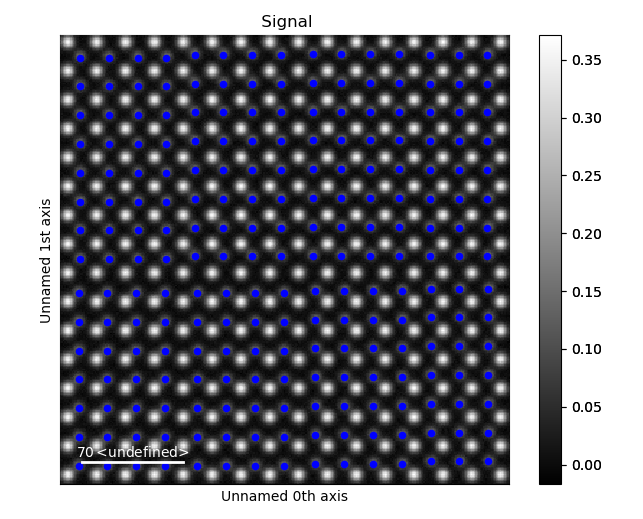## Plot the Lattice Strain Map

By inputting the calculated or theoretical atom plane separation distance as the `theoretical_value` parameter in `temul.topotem.polarisation.get_strain_map()` below, we can plot a strain map. The distance l is calculated as the distance between each atom plane in the given zone axis. More details on this can be found on the Atomap website.

```>>> theor_val = 1.9
>>> strain_map = tml.get_strain_map(sublatticeB, zone_axis_index=0,
...            units=units, sampling=sampling, theoretical_value=theor_val)
```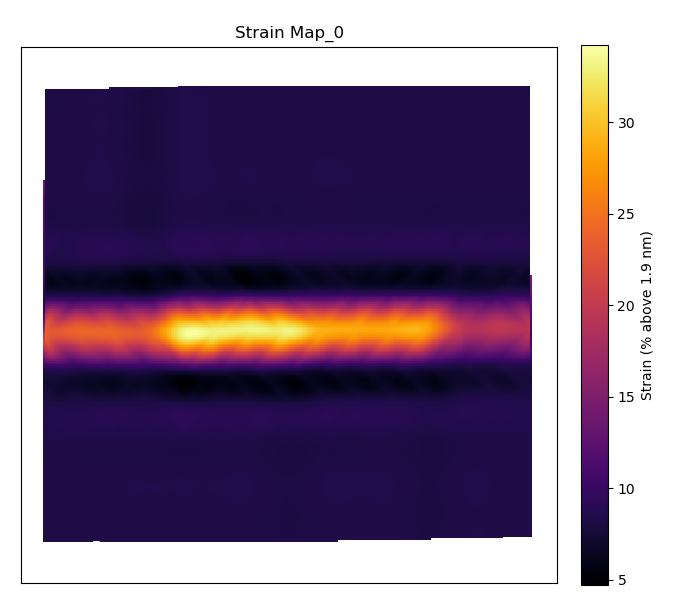The outputted `strain_map` is a Hyperspy Signal2D. To learn more what can be done with Hyperspy, read their documentation!

Setting the `filename` parameter to any string will save the outputted plot and the .hspy signal (Hyperspy’s hdf5 format). This applies to all structure maps discussed in this tutorial.

Setting `return_x_y_z=False` will return the strain map along with the x and y coordinates along with their corresponding strain values. One can then use these values externally, e.g., create a matplotlib tricontour plot). This applies to all structure maps discussed in this tutorial.

## Plot the Lattice Atom Rotation Map

The `temul.topotem.polarisation.rotation_of_atom_planes()` function calculates the angle between successive atoms and the horizontal for all atoms in the given zone axis. See Atomap for other options.

```>>> degrees=True
>>> rotation_map = tml.rotation_of_atom_planes(sublatticeB, 0,
...                     units=units, sampling=sampling, degrees=degrees)

'''
Use `angle_offset` to effectively change the angle of the horizontal axis
when calculating angles. Useful when the zone is not perfectly on the horizontal.
'''

>>> angle_offset = 45
>>> rotation_map = tml.rotation_of_atom_planes(sublatticeB, 0,
...                     units=units, sampling=sampling, degrees=degrees,
...                     angle_offset=angle_offset, title='Offset of 45, Index')
```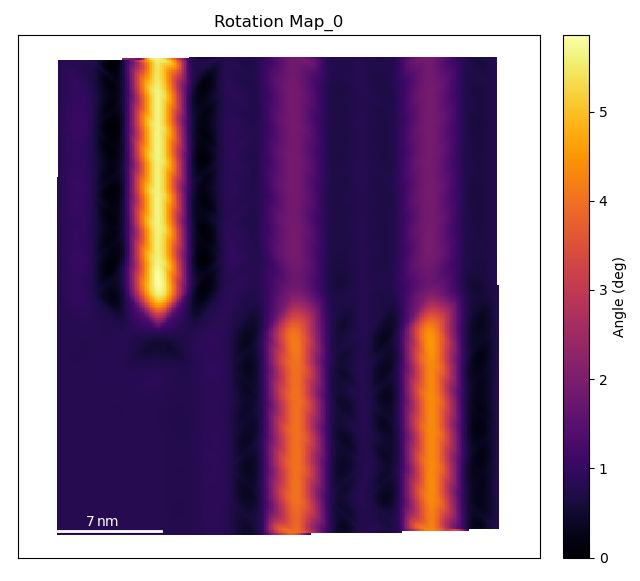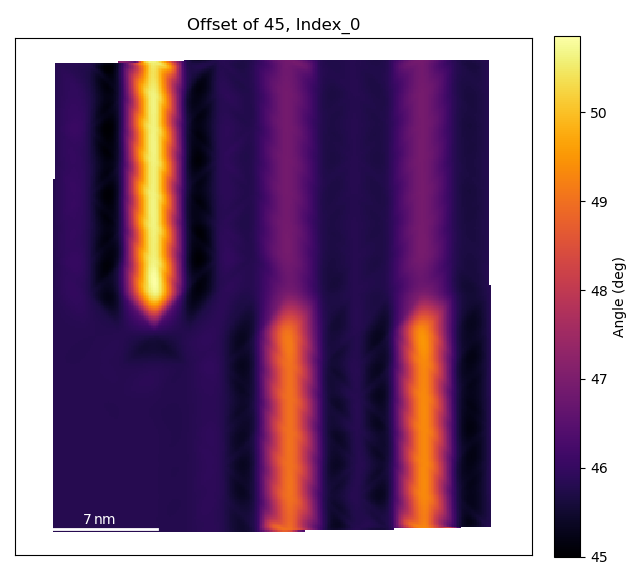## Plot the c/a Ratio

Using the `temul.topotem.polarisation.ratio_of_lattice_spacings()` function, we can visualise the ratio of two sublattice zone axes. Useful for plotting the c/a Ratio.

```>>> ratio_map = tml.ratio_of_lattice_spacings(sublatticeB, 0, 1,
...                 units=units, sampling=sampling)
```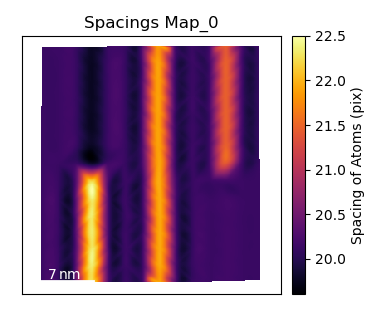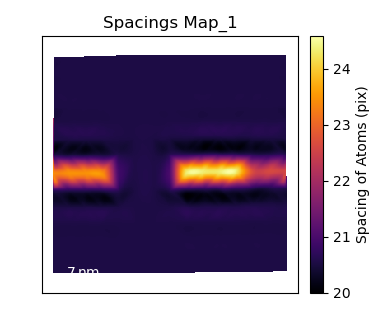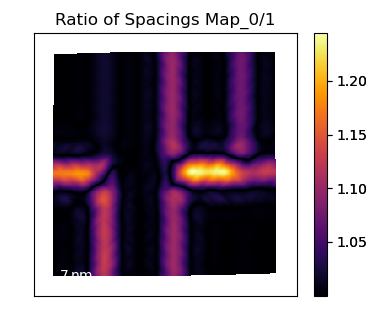One can also use `ideal_ratio_one=False` to view the direction of tetragonality.